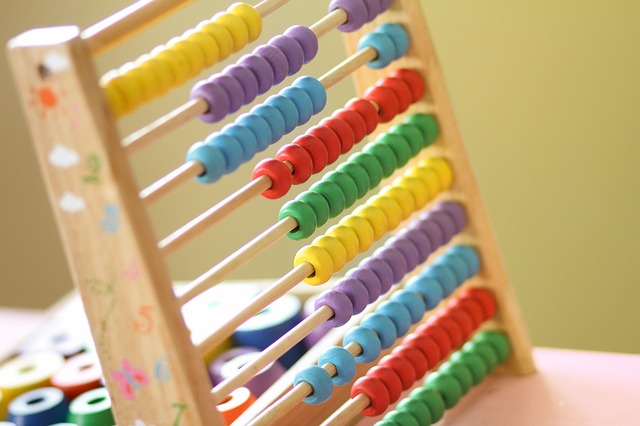Calculators are one of the first electronic devices that started being used by students. While there are a lot of reasons to debate over the use of calculators by students, calculators are still considered to be tools for a student’s academic success. This article describes the reasons for using a calculator by students and the benefits they can derive from its use. Let’s take a look at the benefits of using a calculator:

## Calculators help in illustrating concepts

Being able to relate the theoretical concepts of maths with a practical approach helps in understanding them better. Calculators help students in recognizing the patterns of mathematical problems and enhances the learning speed of students. This is especially beneficial in the study of Algebra and Linear Equations.

## Calculators can help in solving real-world applications

When students are in need of measurements for dimensions and application for concepts of area and volume, they can use a calculator. However, in the real world, these are not measured in whole numbers. To begin with, students should work with whole numbers. Then they can start working with decimals and fractions. The use of calculators can help with this whole process and help them understand the usage of these in the real world.

## Calculators improve your estimation skills

Calculators can enhance your estimation skills. While solving a measurement problem where the distances are not exact, the calculator can help you with the answer. When using calculators, estimate the answers first before doing calculations on the calculator. This will improve your estimation skills. With regular practice, you will find your estimation skill becoming better and better.